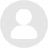# 小孩子口算提速有什么方法？在哪儿可以学？

## 小孩子口算提速有什么方法？在哪儿可以学？1.多练，这毫无疑问。

2.练的时候，分科推进，即个位与个位，个位与十位，各数相错几率差不多，不熟的样式多加些题。

3.游戏一，突然问，练脑子应急反映，在孩子开心的时候或觉得适合的时候，即别让孩子烦，错了别批评，开个玩笑，对了可大大赞叹，让孩子考考你呗。

4.游戏二，让孩子找数字规律，连加的高斯加法，数字黑洞(上网查)，引导好了孩子会爱上数学，可不仅仅是口算了。5.游戏三，打扑克，扑克的加减乘除玩法。

5.培养孩子口算的兴趣。

6.增强孩子学习的毅力。

7.培养孩子良好的习惯。

8.加强基础口算。

9.注重非智力因素的培养，是纠正孩子计算中错误的途径。

如果早点掌握口算能力，在以后的计算还有数学的学习上就能够快人一步，这是一个很重要的基础训练。

1、口诀

2、例题

1376+98=1474计算方法：1376+100-2

3586+898=4484计算方法：3586+1000-102

5768+9897=15665计算方法：5768+10000-103

1、口诀

2、例题

47+74=121计算方法：（4+7）x11=121

68+86=154计算方法：（6+8）x11=154

58+85=143计算方法：（5+8）x11=143

1、口诀

2、例题

+

———————

1、例题

321-98=223

8135-878=7257

91321-8987=82334

2、总结

1、例题

74-47=27

83-38=45

92-29=63

2、总结

1、例题

936-639=297

723-327=396

873-378=495

2、总结

1、心理方面的原因

2、非智力因素的影响。

1、口算方法归纳总结法

2、练习形式多样法

3、口算练习要做到持之以恒

4、养成良好习惯，保持口算的正确性。

1、记一记，背一背。1+9=109+1=10；2+8=108+2=10；3+7=107+3=10；4+6=106+4=10；5+5=10。（一对一对来记）

2、用“凑十法”口算加法。

（想：8+2=10，2+5=7，10+5=15，所以：8+7=15）。

●●●●●●●●（默记）●●●●●●●）所有的点数就是得数）

3、用“凑十法”口算减法。

01、方法是关键。

02、口算提速的训练要坚持经常。

03、偶尔算错不要责备孩子，找出错误原因，然后改正。###### 叛逆严重 抵触父母###### 厌学抵触 成绩下滑###### 自闭抑郁 拒绝沟通#### 家庭教育困惑TUTOR CONFUSION